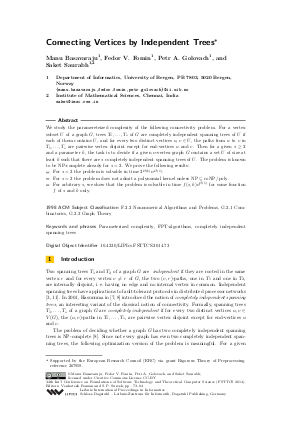Document# Connecting Vertices by Independent Trees

### Authors Manu Basavaraju, Fedor V. Fomin, Petr A. Golovach, Saket Saurabh## File

LIPIcs.FSTTCS.2014.73.pdf
• Filesize: 0.53 MB
• 12 pages

## Cite As

Manu Basavaraju, Fedor V. Fomin, Petr A. Golovach, and Saket Saurabh. Connecting Vertices by Independent Trees. In 34th International Conference on Foundation of Software Technology and Theoretical Computer Science (FSTTCS 2014). Leibniz International Proceedings in Informatics (LIPIcs), Volume 29, pp. 73-84, Schloss Dagstuhl - Leibniz-Zentrum für Informatik (2014)
https://doi.org/10.4230/LIPIcs.FSTTCS.2014.73

## Abstract

We study the paramereteized complexity of the following connectivity problem. For a vertex subset U of a graph G, trees T_1,...,T_s of G are completely independent spanning trees of U if each of them contains U, and for every two distinct vertices u,v in U, the paths from u to v in T_1,...,T_s are pairwise vertex disjoint except for end-vertices u and v. Then for a given s >= 2 and a parameter k, the task is to decide if a given n-vertex graph G contains a set U of size at least k such that there are s completely independent spanning trees of U. The problem is known to be NP-complete already for s=2. We prove the following results: (*) For s=2 the problem is solvable in time 2^{O(k)}*n^{O(1)}. (*) For s=2 the problem does not admit a polynomial kernel unless NP subseteq coNP/poly. (*) For arbitrary s, we show that the problem is solvable in time f(s,k)n^{O(1)} for some function f of s and k only.
##### Keywords
• Parameterized complexity
• FPT-algorithms
• completely independent spanning trees

## Metrics

• Access Statistics
• Total Accesses (updated on a weekly basis)
0